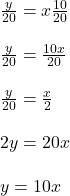## Write a division problem with a 3-digit dividend and divisor of 20 and remainder of 10. pls i need an answer

Question

Write a division problem with a 3-digit dividend and divisor of 20 and remainder of 10. pls i need an answer

in progress 0
5 months 2021-08-09T16:20:07+00:00 1 Answers 23 views 0

y = 10x

(x is a set of odd numbers between 11 and 99)

Step-by-step explanation:

Given;

divisor = 20

remainder, = 10

let the 3 – digits dividend = y

let the quotient = x

Arranging all in mixed fraction;where x = 11, 13, 15, 17, 19 – – – – – – – 99